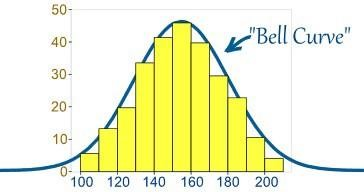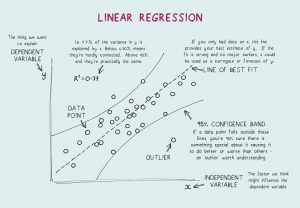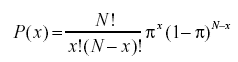HomeBlogData ScienceData Science Interview Questions & Answers – 15 Most Frequently Asked

# Data Science Interview Questions & Answers – 15 Most Frequently Asked

Last updated:
8th Jul, 2019
Views
13 MinsView AllJob interviews are always tricky. To successfully crack an interview, you must possess not only in-depth subject knowledge but also confidence and a strong presence of mind. This is especially true if you are preparing for a Data Science – it puts all your faculties to test!

During a Data Science interview, you’ll have to confront a host of questions spanning from diverse topics ranging from basic Data Science questions to Statistics, Data Analysis, ML, and Deep Learning. But that’s not all – your soft skills (communication, teamwork, and more.) will also be tested.

To ease the preparation process for you, we’ve curated a list of 15 most frequently asked Data Science interview questions. We’ll start with the fundamentals and then move on to the more advanced topics and issues.

So, without further ado, let’s begin!

1. ## What is Data Science? How do Supervised and Unsupervised Machine Learning differ?

In plain words, Data Science is the study of data. It involves the collection of data from disparate sources, storing it, cleaning and organizing it, and analyzing it to uncover meaningful information from it. Data Science uses a combination of Mathematics, Statistics Computer Science, Machine Learning, Data Visualization, Cluster Analysis, and Data Modelling. It aims to gain valuable insights from raw data (both structured and unstructured) and use those insights to influence business and IT strategies positively. Such ideas can help businesses optimize processes, boost productivity and revenue, streamline marketing strategies, enhance customer satisfaction, and much more.

### Supervised and Unsupervised ML differ from each other in the following respects:

• In supervised ML, the input data is labelled. In unsupervised ML, the input data remains unlabeled.
• While supervised ML uses training dataset, unsupervised ML uses the input data set.
• Supervised ML is used for prediction purposes, whereas unsupervised ML is used for analysis purposes.
• Supervised ML enables classification and regression. However, unsupervised ML enables classification, density estimation, and dimension reduction.

Refer to the below-mentioned table to understand the difference between the two:

 Supervised learning Unsupervised learning Labelled input data. Unlabelled input data. Utilises training dataset. Utilises input dataset. Used for prediction. Used for analysis. Two types, namely: Regression Classification Two types, namely: Clustering Association Has known number of classes. Has an unknown number of classes. Use off-line analysis. Use real-time analysis.

It could be a data science exam question that could be asked during interviews or any other exam that you may be having. This makes for the most asked data science entrance test questions.

1. ## Python or R – Which is better for text analytics?

When it comes to text analytics, Python seems like the most suitable option. This is because it comes with the Pandas library that includes user-friendly data structures and high-performance data analysis tools. Also, Python is highly efficient and fast for all kinds of text analytics tasks. As for R, it is best suited for Machine Learning applications.

Refer to the below-mentioned table to understand the difference between these two:

 Python R Integrates system effectively. Specifically designed for statistical analysis. Flexible interact with other programming languages. Less flexible interacting with programming languages. More suitable for deep learning. It is better for data visualisation.

You may respond to the graded questions & answers during the interview or test in the manner given above. It could give leverage over the other candidates. It could also be asked during python exam questions or python interview questions. You should not only be aware of python but also be ready with your responses when asked to compare.

1. ## What are the supported data types in Python?

Python has an array of built-in data types, including:

• Boolean
• Numeric (Integers, Long, Float, Complex)
• Sequences (Lists, Strings, Byte, Tuple)
• Sets
• Mappings (Dictionaries)
• File objects

1. ## What are the different classification algorithms?

The pivotal classification algorithms are linear classifiers (logistic regression, Naive Bayes classifier), decision trees, boosted trees, random forest, SVM, kernel estimation, neural networks, and nearest neighbor.

Logistic regression: It is a linear classification model that is used to predict the probability (p) of an event occurrence.

K- Nearest Neighbours: It is a non-linear classifier that is useful in predicting the class to which new test data belongs. The number of data points in each category is counted.

Support Vector Machine (SVM): The line is drawn equidistant from both sets. It is used as a linear or non-linear classifier that is used on the kernel that is used.

Naive Bayes Classifier: It works on the Bayes theorem. All the features are independent of one another that contributes equally to the outcome.

Decision Tree Classification: It is the most powerful classifier. Based on this tree, splits are created to differentiate classes in the given original dataset.

1. ## What is Normal Distribution?

Usually, data is distributed in various ways either with a bias to the left or the right or in a few circumstances, it may become jumbled up. However, there might be instances where the data is distributed around a central value without any bias to the left or right, thereby attaining a normal distribution in the form of a bell-shaped curve.Source

The curve depicts the distribution of random variables in the form of a symmetrical bell-shaped curve.

## Explore our Popular Data Science Courses

 Executive Post Graduate Programme in Data Science from IIITB Professional Certificate Program in Data Science for Business Decision Making Master of Science in Data Science from University of Arizona Advanced Certificate Programme in Data Science from IIITB Professional Certificate Program in Data Science and Business Analytics from University of Maryland Data Science Courses

1. ## What’s the importance of A/B Testing?

A/B testing is a statistical hypothesis testing for random experimentation involving two variables – A and B. A/B Testing helps identify any changes or alterations made to the web page to maximize the outcome of interest. It is an excellent method to determine the best online promotional and marketing strategies for businesses.

upGrad’s Exclusive Data Science Webinar for you –

Transformation & Opportunities in Analytics & Insights

Benefits of A/B Testing are as follows:

•  Conversion increment.
• Reduction in risk.
• Easy analysis.
• Higher value.
• Enhance user engagement.
• Improved content management.
1. ## What is Selection Bias?

Selection Bias is an ‘active’ error that occurs when the researcher decides the samples that are going to be studied. In this case, the sample data is collected and prepared for data modelling, but it bears such characteristics that are not the true representative of the future population of cases the model will consider. Selection bias takes place when a subset of the sample data is systematically chosen and included/excluded from data analysis. There are three different types of Selection Bias:

## Top Data Science Skills to Learn

 Top Data Science Skills to Learn 1 Data Analysis Course Inferential Statistics Courses 2 Hypothesis Testing Programs Logistic Regression Courses 3 Linear Regression Courses Linear Algebra for Analysis

• ### Sampling bias:

A systematic error that occurs when a non-random sample of a data set causes some members of the data set to be less likely included in the study, thereby leading to a biased sample.

• ### Time interval:

It occurs when a data analysis trial is terminated early at an extreme value. However, the extreme value can be attained more likely by the variable bearing the largest variance (even if all variables possess a similar mean).

• ### Attrition:

It occurs due to attrition discounting, or the loss of participants during a trial that was terminated before completion.

1. ## What is a Linear Regression? What are the assumptions required for linear regression?

Linear Regression is a statistical tool used for predictive analysis. In this method, the score of a variable (say Y) is predicted from the score of another variable (say X). Here, Y is the criterion variable, whereas X is the predictor variable.

Properties of linear regression:

• Line reduces the sum of squared differences.
• The regression passes through the mean of X and Y variables.
• It has the ability to work with any kind of dataset.

In Linear Regression, there are four fundamental assumptions:

• A linear relationship exists between the dependent variables and the regressors. So, the data model created will be in sync with the data.
• The residuals of the data are independent of one another and to be distributed.
• There is minimal multi-collinearity between explanatory variables.
• There is ‘homoscedasticity’ which means that the variance around the regression line is the same for all values of the predictor variable.1. ## What is Cross-validation?

Cross-validation is a model validation procedure used for. The aim here is to term the validation data set to test the model in the training phase to limit problems like overfitting and of course, determine how the model will generalize to an independent data set.

Cross-validation (CV) is a model validation technique employed to test the effectiveness of machine learning models. It is also a re-sampling method used to evaluate a model in case of limited data. In the cross-validation method, a portion of data is set aside for testing and validation and is used to determine how the outcomes of statistical analysis will generalize to an Independent dataset.

Cross validation gives a better accurate estimate of out- of sample accuracy. It works as a resampling procedure that is used to estimate the performance of machine learning models.

1. ## What is the Binomial Probability Formula?

The binomial probability distribution takes into consideration the probabilities of each of the possible numbers of successes out of N number of trials for independent events, each having the probability of occurrence of π (pi). The formula for a binomial probability distribution is:1. ## What is the difference Univariate, Bivariate, and Multivariate analysis?

Univariate analysis refers to the descriptive statistical analysis technique that can be differentiated based on the number of variables involved at a particular point of time (for instance, pie charts depicting the sales of a product in a specific territory). Contrary to this, the bivariate analysis aims to understand and determine the difference between two variables at a time as in a scatterplot (for example, the relationship between the sale volume and spending).

The multivariate analysis involves the study of more than two variables to understand the effect of the variables on the responses/outcomes.

Refer to the below- mentioned table to understand the differences:

 Univariate Bivariate Multivariate Summarises only one variable at a time. Compares two variables. Compares more than two variables. Does not deal with causes or relationships. Deals with causes or relationships. Deals with causes or relationships. Does not contain any independent variable. Contains only one dependent variable. Contains more than one dependent variable. It is used for describing. It is used for explaining. It is used to study the relationships among P attributes.
1. ## What are Artificial Neural Networks?

In plain terms, Artificial Neural Networks (ANN) refers to a computing system designed after the human brain. Just as the human brain, ANNs are composed numerous simple processing elements, known as artificial neurons whose functionality is inspired by the neurons in animal species. ANNs can learn through experience and can adapt to the changing input so that the network can generate the best possible result without having to redesign the output criteria.

• It is alter to unknown conditions.
• It is a powerful technology.
• It can be used to model difficult functions.
• It has the ability to get imposed on any application.
1. ## What are Recurrent Neural Networks (RNNs)?

A recurrent neural network (RNN) is a type of artificial neural network in which nodal connections result in a directed graph along a temporal sequence, thereby exhibiting temporal dynamic behaviour. To understand RNN, you must first understand the workings of feedforward nets. While feedforward networks channel information in a straight line (without touching the same node twice), recurrent neural networks cycles information through a loop-like process. Contrary to feedforward neural nets, RNNs can use their internal memory to process sequences of inputs. Hence, RNNs are best suited for tasks that are unsegmented or connected, like handwriting recognition and speech recognition.

Advantages of RNN are as follows:

• Can be used to process any length input.
• The model size cannot be used to increase for longer input.
• The weights are shared across timesteps.
1. ## What is Back Propagation?

Backpropagation refers to a supervised learning algorithm that is used for training multilayer neural networks. Through backpropagation, an error can be moved from an end of the network to all weights inside the network, thereby allowing efficient computation of the gradient. It seeks out the minimum value of the error function in weight-space using the gradient descent technique. The weights that minimize the error function is regarded as the solution to the learning problem.

• Backpropagation involved the following steps:
• Forward propagation of training data.
• Compute derivatives using output and target.
• Back Propagate for computing derivative of the error.
• Use previously calculated derivatives for output.
• Calculating the updated weight value and updating the weights.

To understand Gradient Descent, you must first understand what a gradient is. A gradient is a measure of how much the output of a particular function changes in relation to a minor change in the inputs. It measures the change in all the weights in response to a change in error. So, in other words, a gradient is the slope of a function.

Gradient descent is an optimization algorithm that helps find the values of parameters (coefficients) of a function (f) that minimizes a cost function (cost). It is best suited for instances when the parameters cannot be calculated analytically.

## Read our popular Data Science Articles

 Data Science Career Path: A Comprehensive Career Guide Data Science Career Growth: The Future of Work is here Why is Data Science Important? 8 Ways Data Science Brings Value to the Business Relevance of Data Science for Managers The Ultimate Data Science Cheat Sheet Every Data Scientists Should Have Top 6 Reasons Why You Should Become a Data Scientist A Day in the Life of Data Scientist: What do they do? Myth Busted: Data Science doesn’t need Coding Business Intelligence vs Data Science: What are the differences?

## Conclusion

On a concluding note, you must know that there’s no single or best way to prepare for an interview. It’s all about your knowledge base, your confidence and approach, and a little luck. While these are just a handful of Data Science questions, we do hope that this gives you a rough idea about the kind of questions you can be asked in a Data Science interview. That said, prepare well, and all the best for your endeavors!

Learn data science courses from the World’s top Universities. Earn Executive PG Programs, Advanced Certificate Programs, or Masters Programs to fast-track your career.#### Abhinav Rai

Blog Author
Abhinav is a Data Analyst at UpGrad. He's an experienced Data Analyst with a demonstrated history of working in the higher education industry. Strong information technology professional skilled in Python, R, and Machine Learning.
Get Free Consultation

Select CourseSelectBy clicking 'Submit' you Agree to

#### Data Science Skills to Master

1In a data science interview, how many rounds are there?

One or two rounds of programming interviews may be required, but this is entirely dependent on the company for which you are applying. Some firms make the interview process last up to six rounds. You can prepare your responses for each question by researching the most frequently asked questions, making a list of the most common and tough questions, and then analyzing those questions before your interview.

2What are the qualities that data science interviewers look for?

To ace a data science interview, you'll need to know a lot about arithmetic, statistics, programming languages, business intelligence fundamentals, and, of course, machine learning techniques. You'll most certainly be asked to demonstrate how your data abilities relate to company choices and strategy. In today's market, almost every data science job requires a coding interview. The role of data scientists includes releasing production code, such as data pipelines and machine learning models, at many firms. For projects of this nature, strong programming abilities are also required, so you can expect some SQL and Python questions too in the interview.

3Can I get a data scientist job through LinkedIn?

4What are the four pillars of data science?

The four pillars of data science include: a.) Collection, b.) Exploration, c.) Visualisation, d.) Modelling

5What are the three main concepts of data science?

The three main concepts of data science include: a.) Data design, b.) Collection, c.) Analysis

6What are the five P’s of data science?

The five P’s of data science are: a.) Purpose, b.) People, c.) Processes, d.) Platforms, e.) Programmability

7What are the top three skills that you need to be a data analyst?

The top three skills of a data analyst include: a.) Econometrics, b.) SQL, c.) Statistical Programming

## Suggested Blogs

294665
Summary: In this Article, you will learn about 4 Types of Data Qualitative Data Type Nominal Ordinal Quantitative Data Type Discrete Continuous R